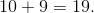# Common Core: 1st Grade Math : 11 to 19 are Composed of a Ten and One, Two, Three, Four, Five, Six, Seven, Eight, or Nine Ones: CCSS.MATH.CONTENT.1.NBT.B.2.B

## Example Questions

← Previous 1

### Example Question #1 : 11 To 19 Are Composed Of A Ten And One, Two, Three, Four, Five, Six, Seven, Eight, Or Nine Ones: Ccss.Math.Content.1.Nbt.B.2.B

What number is ten and one?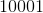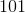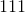Explanation:

Ten and one means the same thing as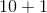. When we add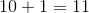.

### Example Question #2 : 11 To 19 Are Composed Of A Ten And One, Two, Three, Four, Five, Six, Seven, Eight, Or Nine Ones: Ccss.Math.Content.1.Nbt.B.2.B

What number is ten and three?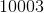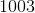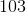Explanation:

Ten and three means the same thing as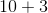. When we add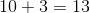.

### Example Question #3 : 11 To 19 Are Composed Of A Ten And One, Two, Three, Four, Five, Six, Seven, Eight, Or Nine Ones: Ccss.Math.Content.1.Nbt.B.2.B

What number is ten and two?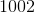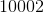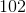Explanation:

Ten and two means the same thing as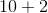. When we add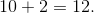### Example Question #4 : 11 To 19 Are Composed Of A Ten And One, Two, Three, Four, Five, Six, Seven, Eight, Or Nine Ones: Ccss.Math.Content.1.Nbt.B.2.B

What number is ten and four?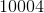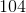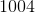Explanation:

Ten and four means the same thing as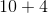. When we add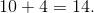### Example Question #1 : 11 To 19 Are Composed Of A Ten And One, Two, Three, Four, Five, Six, Seven, Eight, Or Nine Ones: Ccss.Math.Content.1.Nbt.B.2.B

What number is ten and five?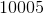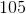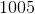Explanation:

Ten and five means the same thing as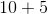. When we add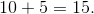### Example Question #6 : 11 To 19 Are Composed Of A Ten And One, Two, Three, Four, Five, Six, Seven, Eight, Or Nine Ones: Ccss.Math.Content.1.Nbt.B.2.B

What number is ten and six?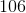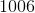Explanation:

Ten and six means the same thing as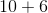. When we add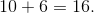### Example Question #1 : 11 To 19 Are Composed Of A Ten And One, Two, Three, Four, Five, Six, Seven, Eight, Or Nine Ones: Ccss.Math.Content.1.Nbt.B.2.B

What number is ten and seven?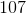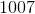Explanation:

Ten and seven means the same thing as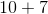. When we add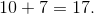### Example Question #2 : 11 To 19 Are Composed Of A Ten And One, Two, Three, Four, Five, Six, Seven, Eight, Or Nine Ones: Ccss.Math.Content.1.Nbt.B.2.B

What number is ten and eight?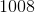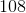Explanation:

Ten and eight means the same thing as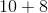. When we add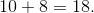### Example Question #1 : 11 To 19 Are Composed Of A Ten And One, Two, Three, Four, Five, Six, Seven, Eight, Or Nine Ones: Ccss.Math.Content.1.Nbt.B.2.B

What number is ten and zero?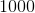Explanation:

Ten and zero means the same thing as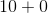. When we add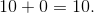### Example Question #10 : 11 To 19 Are Composed Of A Ten And One, Two, Three, Four, Five, Six, Seven, Eight, Or Nine Ones: Ccss.Math.Content.1.Nbt.B.2.B

What number is ten and nine?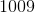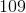Ten and nine means the same thing as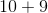. When we add